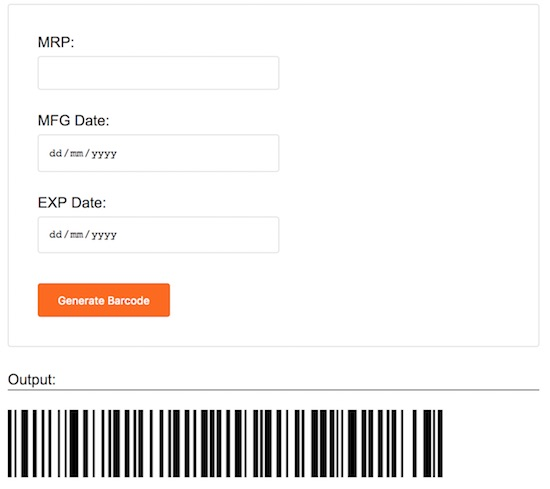How to Create Barcode Generator using PHP

Barcode is a machine-readable ident bundled with data about an entity. It is particularly used to store product-related data like price, code, manufacturing date, and similar data.

In this tutorial, we are going to create PHP code for generating barcodes. I used the tc-lib-barcode library for creating barcodes using PHP.

If you are looking for a QR code generator, in a previous tutorial, we have seen how to create QR code using this library.

In this example, the product price, MFG and EXP dates are bundled in the form of Barcode. By scanning the generated barcode image with a barcode reader app, these data can be revealed.

We can add this PHP code for a shopping cart application to generate the barcode for products as unique identification reference.

An HTML form is used to collect the product data. On submitting this form, the data are validated and bundled in a right format. The formatted data will be passed to the library function to generate the barcode.Download the library source code from the GitHub to execute this example.

HTML Form to getting Product Data

This HTML form contains inputs for getting the product MRP, MFG and EXP date. These data are validated using JavaScript on the form submit.

If anything goes wrong while validation, the function will return false and display the error below this form. On successful validation, the form data will be posted to PHP code to create the barcode.

<form method="post" name="frmBarcodeGenerator" id="frmBarcodeGenerator"
onSubmit="return validate();">
<div class="form-row">
MRP:
<div>
<input type="text" name="mrp" id="mrp" class="input-field" />
</div>
</div>
<div class="form-row">
MFG Date:
<div>
<input type="date" name="mfg_date" id="mfg_date"
class="input-field" />
</div>
</div>
<div class="form-row">
EXP Date:
<div>
<input type="date" name="exp_date" id="exp_date"
class="input-field" />
</div>
</div>

<div>
<input type="submit" name="generate" class="submit-button"
value="Generate Barcode" />
</div>
</form>

This is the JavaScript contains the validation function. It checks the not-empty validation on each form data and also checks if the product MRP is numeric.

<script>
function validate() {
var valid = true;
var message;

\$("#validation-info").hide();
\$("#validation-info").html();
if(\$("#mrp").val() == "") {
message = "All fields are required";
valid = false;
} else if(!\$.isNumeric(\$("#mrp").val())) {
message = "MRP should be in numbers";
valid = false;
} else if(\$("#mfg_date").val() == "") {
message = "All fields are required";
valid = false;
} else if(\$("#exp_date").val() == "") {
message = "All fields are required";
valid = false;
}
if(valid == false) {
\$("#validation-info").show();
\$("#validation-info").html(message);
}
return valid;
}
</script>

PHP Code Forms Product Data to Generate BarCode

In this PHP code, the tc-lib-barcode library autoload file is included at the beginning. Then the form data is received by using the POST request data array. The MFG and EXP dates are converted into a timestamp.

Then, the product MRP and MFG/EXP timestamps are bundled into a string format. This formatted product data will be passed to the getBarcodeObj. This function will return barcode object based on the type specified.

With the reference of the barcode object, the barcode png image will be created and displayed to the browser. In a previous tutorial, we have seen how to create an image dynamically by using PHP.

<?php
if (! empty(\$_POST["generate"])) {
\$barcode = new \Com\Tecnick\Barcode\Barcode();
\$targetPath = "barcode/";

if (! is_dir(\$targetPath)) {
mkdir(\$targetPath, 0777, true);
}
\$MRP = \$_POST["mrp"];
\$MFGDate = strtotime(\$_POST["mfg_date"]);
\$EXPDate = strtotime(\$_POST["exp_date"]);
\$productData = "098{\$MRP}10{\$MFGDate}55{\$EXPDate}";
\$barcode = new \Com\Tecnick\Barcode\Barcode();
\$bobj = \$barcode->getBarcodeObj('C128C', "{\$productData}", 450, 70, 'black', array(
0,
0,
0,
0
));

\$imageData = \$bobj->getPngData();
\$timestamp = time();

file_put_contents(\$targetPath . \$timestamp . '.png', \$imageData);
?>